# 【车牌识别】基于matlab投影模板匹配车牌识别【含Matlab源码 1359期】

1369

## 一、车牌识别简介

2车牌识别系统的设计 2.1车牌定位 车牌定位采用颜色特征提取，一般的车牌区域都具有很明显的特点，中国的车牌以蓝底白字居多。传统的方法一般根据车牌的色彩特征，彩色像素点统计的方法分割出合理的车牌区域假设经相机采集包含车牌的RGB图像， 水平方向记为y，垂直方向记为x。首先，确定车牌各分量分别对应的颜色范围。其次，计算水平方向上对应的像素数量和车牌的合理面积。然后，计算分割的水平方向区域中垂直方向上该颜色范围内白的像素数量，并为定位设置合理的阈值。最后，根据对应方向的范围确定车牌区域。但是这种方法的准确率较低，本文采用文献提出的颜色特征提取及二值化归类方法进行算法改进，计算方法如公式(4)所示。图5为定位后的车牌。 其中的蓝色特征可用式(4)表示：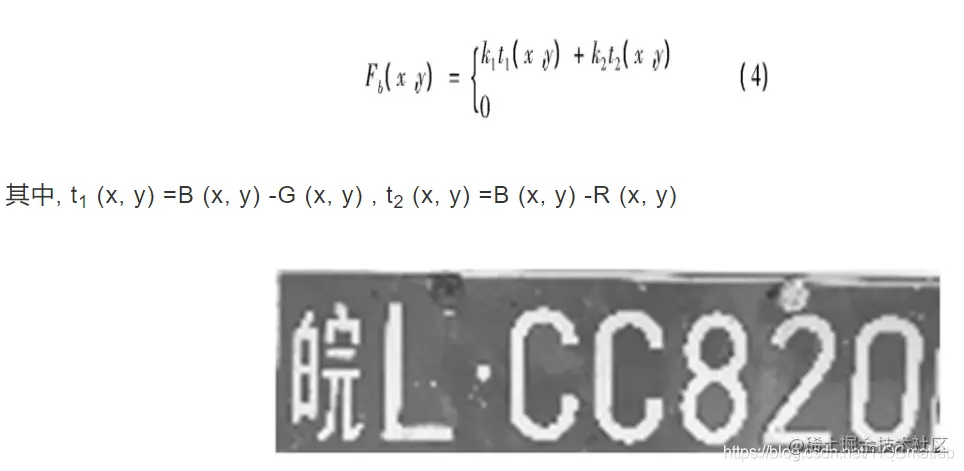图5 车牌定位图 2.2车牌字符识别 在字符识别前，先对车牌字符分割。字符分割采用阈值分割，主要包含两个步骤【7]。 (1)确定所需要进行分割的阈值。 (2)将阈值与每个点的灰度值对照，以达到分割目的。 将分割后的图像进行归一化处理，可以有效地将字符图像的大小进行缩放以得到大小一致的字符图像，便于后续的字符识别。 模板匹配一般是数字图像处理中最常使用的识别方法之一，先建立模板库，再将字符输入到模板中寻找与之最佳匹配的模板字符。 模板图像大小为20×40，模板库由数字0-9，32个省份的简称汉字和大写英文字母(其中O一般不用作车牌字母)三部分组成。模板的部分图像如图6所示。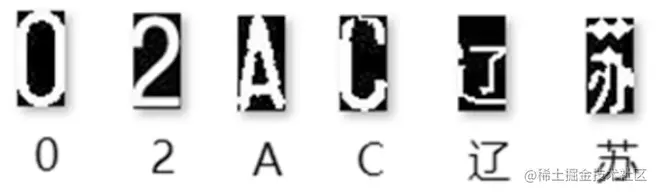图6 模板库部分图片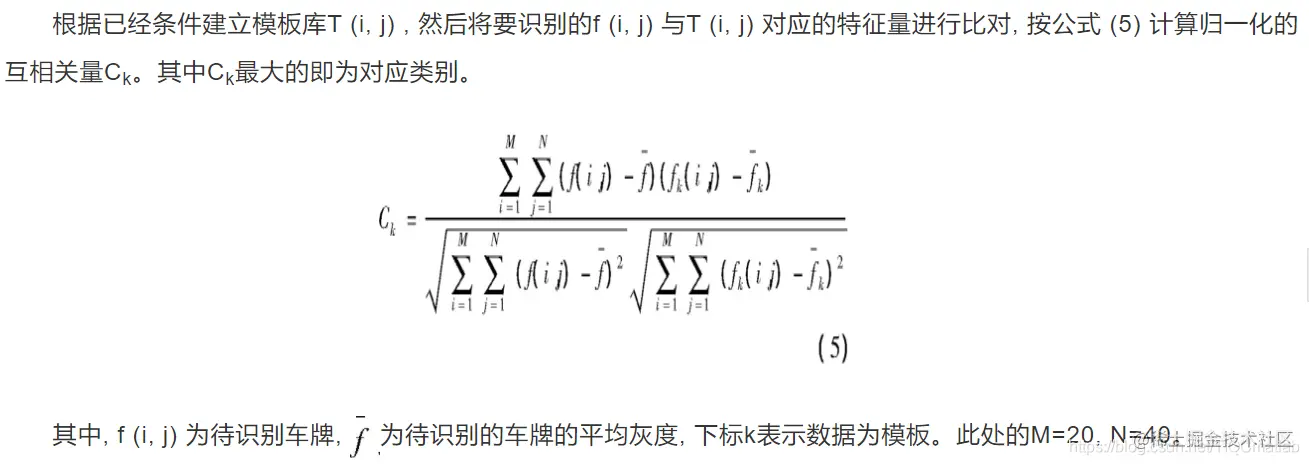## 二、部分源代码

``````%%
clear;
close all;
clc;

%% 自动弹出提示框读取图像
[filename filepath] = uigetfile('.jpg', '输入一个需要识别的图像');
file = strcat(filepath, filename);
figure;
imshow(img);
title('车牌图像');

%% 灰度处理
img1 = rgb2gray(img);    % RGB图像转灰度图像
figure;
subplot(1, 2, 1);
imshow(img1);
title('灰度图像');
subplot(1, 2, 2);
imhist(img1);
title('灰度处理后的灰度直方图');

%% 边缘提取
img4 = edge(img1, 'roberts', 0.15, 'both');
figure('name','边缘检测');
imshow(img4);
title('roberts算子边缘检测');

%% 图像腐蚀
se=[1;1;1];
img5 = imerode(img4, se);
figure('name','图像腐蚀');
imshow(img5);
title('图像腐蚀后的图像');

%% 平滑图像，图像膨胀
se = strel('rectangle', [30, 30]);
img6 = imclose(img5, se);
figure('name','平滑处理');
imshow(img6);
title('平滑图像的轮廓');

%% 从图像中删除所有少于2200像素8邻接
img7 = bwareaopen(img6, 2200);
figure('name', '移除小对象');
imshow(img7);
title('从图像中移除小对象');

%% 切割出图像
[y, x, z] = size(img7);
img8 = double(img7);    % 转成双精度浮点型

% 车牌的蓝色区域
% Y方向
blue_Y = zeros(y, 1);
for i = 1:y
for j = 1:x
if(img8(i, j) == 1) % 判断车牌位置区域
blue_Y(i, 1) = blue_Y(i, 1) + 1;    % 像素点统计
end
end
end

% 找到Y坐标的最小值
img_Y1 = 1;
while (blue_Y(img_Y1) < 5) && (img_Y1 < y)
img_Y1 = img_Y1 + 1;
end

% 找到Y坐标的最大值
img_Y2 = y;
while (blue_Y(img_Y2) < 5) && (img_Y2 > img_Y1)
img_Y2 = img_Y2 - 1;
end

% x方向
blue_X = zeros(1, x);
for j = 1:x
for i = 1:y
if(img8(i, j) == 1) % 判断车牌位置区域
blue_X(1, j) = blue_X(1, j) + 1;
end
end
end

% 找到x坐标的最小值
img_X1 = 1;
while (blue_X(1, img_X1) < 5) && (img_X1 < x)
img_X1 = img_X1 + 1;
end

% 找到x坐标的最小值
img_X2 = x;
while (blue_X(1, img_X2) < 5) && (img_X2 > img_X1)
img_X2 = img_X2 - 1;
end

% 对图像进行裁剪
img9 = img(img_Y1:img_Y2, img_X1:img_X2, :);
figure('name', '定位剪切图像');
imshow(img9);
title('定位剪切后的彩色车牌图像')

% 保存提取出来的车牌图像
imwrite(img9, '车牌图像.jpg');

%% 对车牌图像作图像预处理

% 转换成灰度图像
plate_img1 = rgb2gray(plate_img);    % RGB图像转灰度图像
figure;
subplot(1, 2, 1);
imshow(plate_img1);
title('灰度图像');
subplot(1, 2, 2);
imhist(plate_img1);
title('灰度处理后的灰度直方图');

% 直方图均衡化
plate_img2 = histeq(plate_img1);
figure('name', '直方图均衡化');
subplot(1,2,1);
imshow(plate_img2);
title('直方图均衡化的图像');
subplot(1,2,2);
imhist(plate_img2);
title('直方图');

% 二值化处理
plate_img3 = im2bw(plate_img2, 0.76);
figure('name', '二值化处理');
imshow(plate_img3);
title('车牌二值图像');

% 中值滤波
plate_img4 = medfilt2(plate_img3);
figure('name', '中值滤波');
imshow(plate_img4);
title('中值滤波后的图像');

%% 进行字符识别
plate_img5 = my_imsplit(plate_img4);
[m, n] = size(plate_img5);

s = sum(plate_img5);    %sum(x)就是竖向相加，求每列的和，结果是行向量;
j = 1;
k1 = 1;
k2 = 1;
while j ~= n
while s(j) == 0
j = j + 1;
end
k1 = j;
while s(j) ~= 0 && j <= n-1
j = j + 1;
end
k2 = j + 1;
if k2 - k1 > round(n / 6.5)
[val, num] = min(sum(plate_img5(:, [k1+5:k2-5])));
plate_img5(:, k1+num+5) = 0;
end
end

y1 = 10;
y2 = 0.25;
flag = 0;
word1 = [];
while flag == 0
[m, n] = size(plate_img5);
left = 1;
width = 0;
while sum(plate_img5(:, width+1)) ~= 0
width = width + 1;
end
if width < y1
plate_img5(:, [1:width]) = 0;
plate_img5 = my_imsplit(plate_img5);
else
temp = my_imsplit(imcrop(plate_img5, [1,1,width,m]));
[m, n] = size(temp);
all = sum(sum(temp));
two_thirds=sum(sum(temp([round(m/3):2*round(m/3)],:)));
if two_thirds/all > y2
flag = 1;
word1 = temp;
end
plate_img5(:, [1:width]) = 0;
plate_img5 = my_imsplit(plate_img5);
end
end

figure;
subplot(2,4,1), imshow(plate_img5);

% 分割出第二个字符
[word2,plate_img5]=getword(plate_img5);
subplot(2,4,2), imshow(plate_img5);
% 分割出第三个字符
[word3,plate_img5]=getword(plate_img5);
subplot(2,4,3), imshow(plate_img5);
% 分割出第四个字符
[word4,plate_img5]=getword(plate_img5);
subplot(2,4,4), imshow(plate_img5);
% 分割出第五个字符
[word5,plate_img5]=getword(plate_img5);
subplot(2,3,4), imshow(plate_img5);
% 分割出第六个字符
[word6,plate_img5]=getword(plate_img5);
subplot(2,3,5), imshow(plate_img5);
% 分割出第七个字符
[word7,plate_img5]=getword(plate_img5);
subplot(2,3,6), imshow(plate_img5);

figure;
subplot(5,7,1),imshow(word1),title('1');
subplot(5,7,2),imshow(word2),title('2');
subplot(5,7,3),imshow(word3),title('3');
subplot(5,7,4),imshow(word4),title('4');
subplot(5,7,5),imshow(word5),title('5');
subplot(5,7,6),imshow(word6),title('6');
subplot(5,7,7),imshow(word7),title('7');

word1=imresize(word1,[40 20]);%imresize对图像做缩放处理，常用调用格式为：B=imresize(A,ntimes,method)；其中method可选nearest,bilinear（双线性）,bicubic,box,lanczors2,lanczors3等
word2=imresize(word2,[40 20]);
word3=imresize(word3,[40 20]);
word4=imresize(word4,[40 20]);
word5=imresize(word5,[40 20]);
word6=imresize(word6,[40 20]);
word7=imresize(word7,[40 20]);

subplot(5,7,15),imshow(word1),title('11');
subplot(5,7,16),imshow(word2),title('22');
subplot(5,7,17),imshow(word3),title('33');
subplot(5,7,18),imshow(word4),title('44');
subplot(5,7,19),imshow(word5),title('55');
subplot(5,7,20),imshow(word6),title('66');
subplot(5,7,21),imshow(word7),title('77');

imwrite(word1,'1.jpg'); % 创建七位车牌字符图像
imwrite(word2,'2.jpg');
imwrite(word3,'3.jpg');
imwrite(word4,'4.jpg');
imwrite(word5,'5.jpg');
imwrite(word6,'6.jpg');
imwrite(word7,'7.jpg');

``````

## 三、运行结果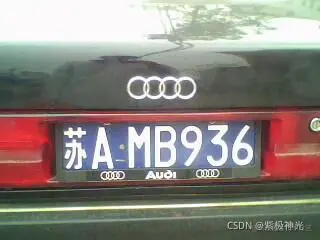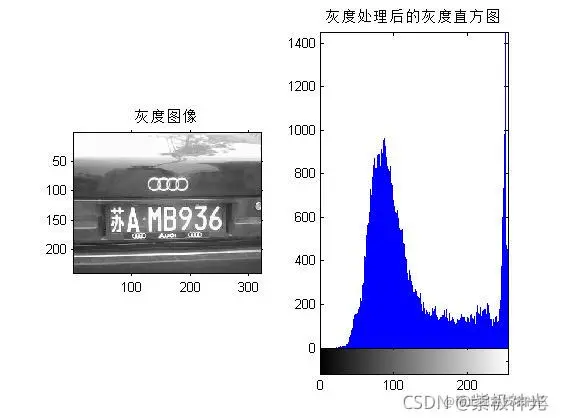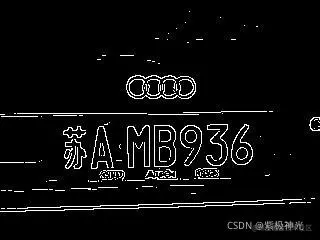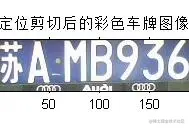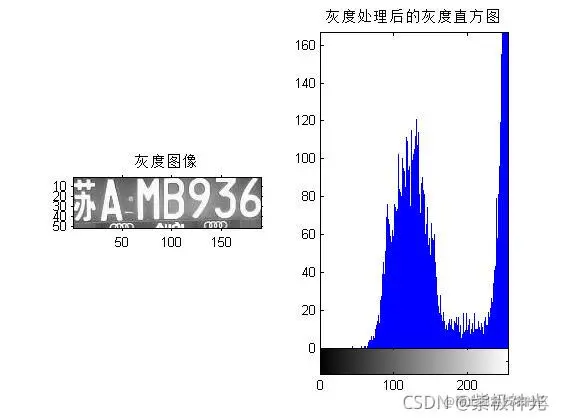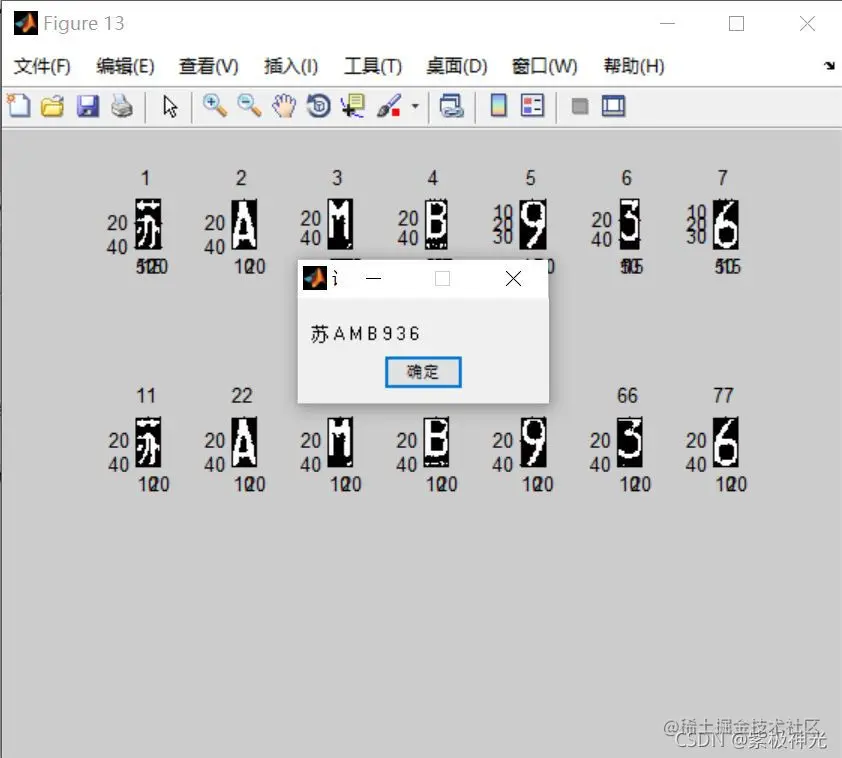## 四、matlab版本及参考文献

1 matlab版本 2014a

2 参考文献  蔡利梅.MATLAB图像处理——理论、算法与实例分析[M].清华大学出版社，2020. 杨丹,赵海滨,龙哲.MATLAB图像处理实例详解[M].清华大学出版社，2013. 周品.MATLAB图像处理与图形用户界面设计[M].清华大学出版社，2013. 刘成龙.精通MATLAB图像处理[M].清华大学出版社，2015. 呙润华，苏婷婷，马晓伟.BP神经网络联合模板匹配的车牌识别系统[J].清华大学学报(自然科学版)，2013，53(9)：1221-1226. 鲁扬.基于BP神经网络的车牌识别算法研究[D].大庆：东北石油大学，2018. 李强,张娟.一种改进的基于模板匹配的污损车牌识别方法[J].智能计算机与应用. 2019,9(03).  梁凯.基于MATLAB的汽车车牌识别系统的设计与实现[D] .哈尔滨：黑龙江大学， 2018. 刘雄飞，朱盛春.车牌字符多特征提取与BP神经网络的识别算法[J].计算机仿真，2014，31(10)：161-164，290.  曾泉， 谭北海.基于SVM和BP神经网络的车牌识别系统[J] .电子科技， 2016， 29(1) ：98-101.